# Extent

## Summary

An extent is a rectangle specified by providing the coordinate of the lower left corner and the coordinate of the upper right corner in map units.

## Discussion

##### Note:

When used as input to ArcGIS Spatial Analyst extension tools—Create Constant Raster, Create Normal Raster, Create Random Raster, Extract By Rectangle, and Topo To Raster—only the XMin, YMin, XMax, and YMax values are used by these tools.

## Syntax

` Extent  ({XMin}, {YMin}, {XMax}, {YMax}, {ZMin}, {ZMax}, {MMin}, {MMax}, {spatial_reference})`
 Parameter Explanation Data Type XMin The extent XMin value. Double YMin The extent YMin value. Double XMax The extent XMax value. Double YMax The extent YMax value. Double ZMin The extent ZMin value. None if no z-value. Double ZMax The extent ZMax value. None if no z-value. Double MMin The extent MMin value. None if no m-value. Double MMax The extent MMax value. None if no m-value. Double spatial_reference The spatial reference of the extent. SpatialReference

## Properties

PropertyExplanationData Type
JSON

Returns a JSON representation of the extent as a string.

##### Tip:

The returned string can be converted to a dictionary using the Python json.loads function.

String
MMax

The extent MMax value. None if no m-value.

Double
MMin

The extent MMin value. None if no m-value.

Double
XMax

The extent XMax value.

Double
XMin

The extent XMin value.

Double
YMax

The extent YMax value.

Double
YMin

The extent YMin value.

Double
ZMax

The extent ZMax value. None if no z-value.

Double
ZMin

The extent ZMin value. None if no z-value.

Double
depth

The extent depth value. None if no depth.

Double
geohash

A geohash string of the extent is returned.

String
geohashCovers

Returns a list of up to the four longest geohash strings that fit within the extent.

String
geohashNeighbors

A list of the geohash neighbor strings for the extent is returned.

String
height

The extent height value.

Double
lowerLeft

The lower left property: A point object is returned.

Point
lowerRight

The lower right property: A point object is returned.

Point
polygon

Returns the extent as a polygon object.

Polygon
spatialReference

The spatial reference of the extent.

SpatialReference
upperLeft

The upper left property: A point object is returned.

Point
upperRight

The upper right property: A point object is returned.

Point
width

The extent width value.

Double

## Method Overview

 Method Explanation contains (second_geometry, {relation}) Indicates if the base geometry contains the comparison geometry. contains is the opposite of within. Only True relationships are shown in this illustration.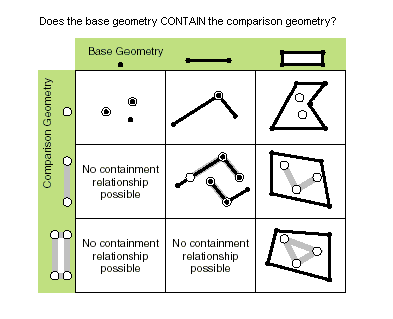crosses (second_geometry) Indicates if the two geometries intersect in a geometry of a lesser shape type. Two polylines cross if they share only points in common, at least one of which is not an endpoint. A polyline and an polygon cross if they share a polyline or a point (for vertical line) in common on the interior of the polygon which is not equivalent to the entire polyline. Only True relationships are shown in this illustration.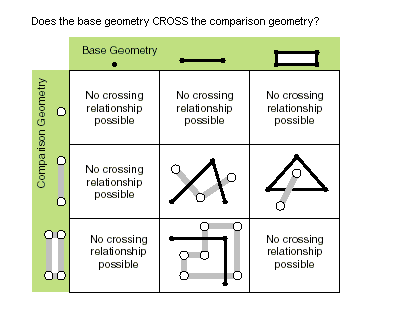disjoint (second_geometry) Indicates if the base and comparison geometries share no points in common. Two geometries intersect if disjoint returns False. Only True relationships are shown in this illustration.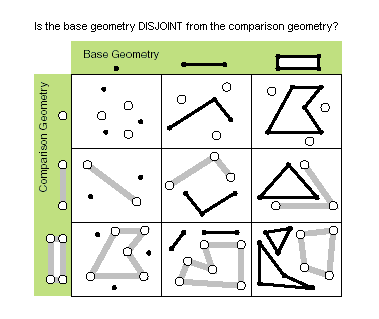equals (second_geometry) Indicates if the base and comparison geometries are of the same shape type and define the same set of points in the plane. This is a 2D comparison only; M and Z values are ignored.Only True relationships are shown in this illustration.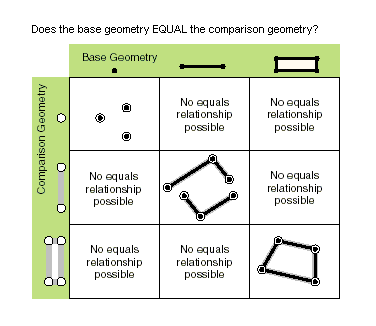overlaps (second_geometry) Indicates if the intersection of the two geometries has the same shape type as one of the input geometries and is not equivalent to either of the input geometries. Only True relationships are shown in this illustration.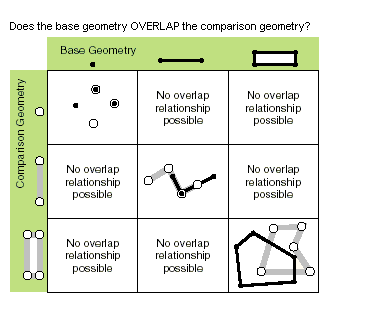projectAs (spatial_reference, {transformation_name}) Projects a geometry and optionally applies a geotransformation. To project, the geometry needs to have a spatial reference, and not have an UnknownCoordinateSystem. The new spatial reference system passed to the method defines the output coordinate system. If either spatial reference is unknown the coordinates will not be changed. The Z- and measure values are not changed by the ProjectAs method. touches (second_geometry) Indicates if the boundaries of the geometries intersect. Two geometries touch when the intersection of the geometries is not empty, but the intersection of their interiors is empty. For example, a point touches a polyline only if the point is coincident with one of the polyline end points. Only True relationships are shown in this illustration.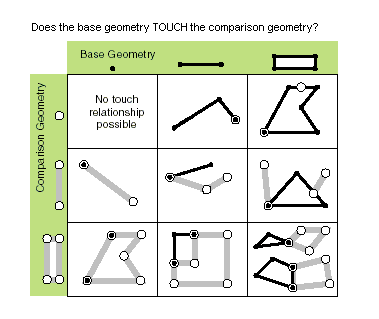within (second_geometry, {relation}) Indicates if the base geometry is within the comparison geometry. within is the opposite operator of contains. Only True relationships are shown in this illustration.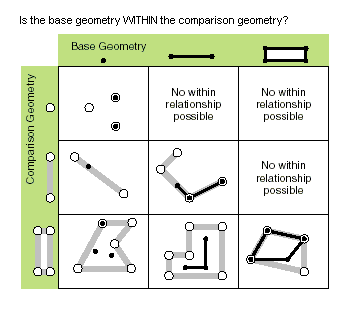The base geometry is within the comparison geometry if the base geometry is the intersection of the geometries and the intersection of their interiors is not empty. within is a Clementini operator, except in the case of an empty base geometry.

## Methods

`contains (second_geometry, {relation})`
 Parameter Explanation Data Type second_geometry A second geometry. Object relation The spatial relationship type.BOUNDARY — Relationship has no restrictions for interiors or boundaries.CLEMENTINI — Interiors of geometries must intersect. Specifying CLEMENTINI is equivalent to specifying None. This is the default.PROPER — Boundaries of geometries must not intersect.(The default value is None) String
Return Value
 Data Type Explanation Boolean A return Boolean value of True indicates this geometry contains the second geometry.
`crosses (second_geometry)`
 Parameter Explanation Data Type second_geometry A second geometry. Object
Return Value
 Data Type Explanation Boolean A return Boolean value of True indicates the two geometries intersect in a geometry of a lesser shape type.
`disjoint (second_geometry)`
 Parameter Explanation Data Type second_geometry A second geometry. Object
Return Value
 Data Type Explanation Boolean A return Boolean value of True indicates that the two geometries share no points in common.
`equals (second_geometry)`
 Parameter Explanation Data Type second_geometry A second geometry. Object
Return Value
 Data Type Explanation Boolean A return Boolean value of True indicates that the two geometries are of the same shape type and define the same set of points in the plane.
`overlaps (second_geometry)`
 Parameter Explanation Data Type second_geometry A second geometry. Object
Return Value
 Data Type Explanation Boolean A return Boolean value of True indicates the intersection of the two geometries has the same dimension as one of the input geometries.
`projectAs (spatial_reference, {transformation_name})`
 Parameter Explanation Data Type spatial_reference The new spatial reference. This can be a SpatialReference object or the coordinate system name. SpatialReference transformation_name The geotransformation name. String
Return Value
 Data Type Explanation Object The projected geometry.
`touches (second_geometry)`
 Parameter Explanation Data Type second_geometry A second geometry. Object
Return Value
 Data Type Explanation Boolean A return Boolean value of True indicates the boundaries of the geometries intersect.
`within (second_geometry, {relation})`
 Parameter Explanation Data Type second_geometry A second geometry. Object relation The spatial relationship type.BOUNDARY — Relationship has no restrictions for interiors or boundaries.CLEMENTINI — Interiors of geometries must intersect. Specifying CLEMENTINI is equivalent to specifying None. This is the default.PROPER — Boundaries of geometries must not intersect.(The default value is None) String
Return Value
 Data Type Explanation Boolean A return Boolean value of True indicates this geometry is contained within the second geometry.

## Code sample

Extent example

Display extent object properties for features.

``````import arcpy

feature_class = 'c:/Data/Florida.gdb/airports'

# Fetch each feature from the cursor and examine the extent properties
for row in arcpy.da.SearchCursor(feature_class, ['SHAPE@', 'CNTY_NAME']):
extent = row.extent
print('Extent of county {}:'.format(row))
print('XMin: {}, YMin: {}'.format(extent.XMin, extent.YMin))
print('XMax: {}, YMax: {}'.format(extent.XMax, extent.YMax))``````

#### Related topics

##### Related topics
1. Extent (Environment setting)